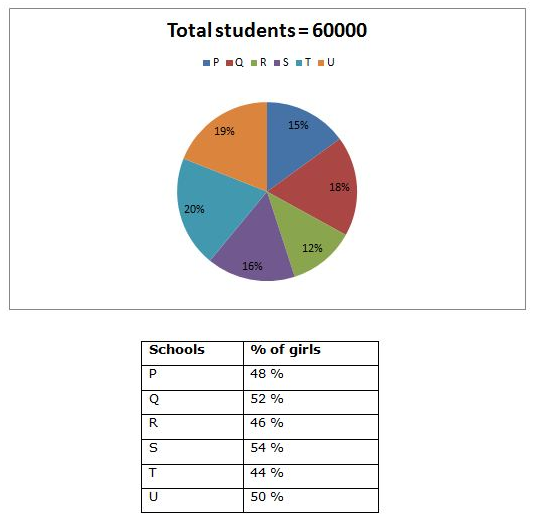# SBI PO Prelims Quantitative Aptitude (Day-12)

Dear Aspirants, Our IBPS Guide team is providing new series of Quantitative Aptitude Questions for SBI PO 2020 so the aspirants can practice it on a daily basis. These questions are framed by our skilled experts after understanding your needs thoroughly. Aspirants can practice these new series questions daily to familiarize with the exact exam pattern and make your preparation effective.

Start Quiz

Ensure Your Ability Before the ExamTake SBI PO 2020 Prelims Free Mock            Test

Application sums

1) A man takes to row 120 km along the stream is one-third of the time taken to row against the stream. Find the speed of the boat in still water if the speed of stream is 8 km/hr?

A) 16 km/hr

B) 20 km/hr

C) 18 km/hr

D) 14 km/hr

E) None of these

2)  An article was sold at a discount of 25 % and there was a profit of 20 %. If the profit earned was Rs. 100 less than the discount offered, then find the selling price of the article?

A) Rs. 600

B) Rs. 700

C) Rs. 750

D) Rs. 650

E) None of these

3) A basket contains 7 White, 5 Black, 2 Pink and 4 Green balls. If three balls picked up random, then find the probability that all three are Green balls?

A) 1/204

B) 15/157

C) 37/218

D) 23/512

E) None of these

4)  5 years ago, the ages of Agila and Hema are in the ratio of 3: 2. Three years ago, Agila’s age is equal to Hema’s age after 2 years. Then find the present age Agila?

A) 20 years

B) 24 years

C) 26 years

D) 18 years

E) None of these

5)  A can do a piece of work in 24 days; B can do it in 30 days. They started the work together but A left 6 days before the completion of the work. The work has finished in?

A) 12 days

B) 15 days

C) 25/3 days

D) 50/3 days

E) None of these

Data interpretation

Directions (06 – 10): Study the following information carefully and answer the given questions.

The following pie chart shows the total number of students in 6 different schools and the table shows the percentage of girls out of the total number of students in the respective schools.6) Find the difference between the total number of boys in School P to that of total number of girls in School R?

A) 1368

B) 1892

C) 1456

D) 1575

E) None of these

7) Find the average number of girls in School P, Q and S together?

A) 5040

B) 5180

C) 4890

D) 4560

E) None of these

8) Find the ratio between the total number of students in School R to that of total number of boys in School T?

A) 8: 7

B) 15: 14

C) 23: 21

D) 32: 29

E) None of these

9) The total number of girls in School U is approximately what percentage of total number of boys in School Q?

A) 110 %

B) 95 %

C) 135 %

D) 70 %

E) 120 %

10) Find the central angle for School T?

A) 720

B) 800

C) 660

D) 840

E) None of these

Directions (1-5) :

Let us take the speed of the boat in still water be x km/hr,

120/(x+8) = (1/3)*(120/(x-8))

3x – 24 = x + 8

2x = 32

X = 16

Speed of the boat in still water = 16 km/hr

Let the cost price be x,

Selling price = (120/100)*x = 6x/5

Marked price*(75/100) = 6x/5

MP = 8x/5

The profit earned was Rs.100 less than the discount,

According to the question,

= > (8x/5 – 6x/5) – (6x/5 – x) = 100

= > 2x/5 – x/5 = 100

= > x/5 = 100

Cost price = Rs. 500

Selling price = 500*(120/100) = Rs. 600

Total Balls = 18

Required Probability = 4C3 / 18C3 = 1/204

5 years ago, Agila: Hema = 3: 2 (3x, 2x)

Present ages of Agila and Hema = 3x + 5, 2x + 5

Three years ago, Agila’s age is equal to Hema’s age after 2 years,

= > 3x + 2 = 2x + 7

= > x = 5

Present age of Agila = 3x + 5 = 20 years

(x – 6)/24 + x/30 = 1

(30x – 180 + 24x)/720 = 1

54x – 180 = 720

54x = 900

X = 900/54 = 50/3 days

Directions (06 – 10):The total number of boys in School P

= > 60000*(15/100)*(52/100) = 4680

The total number of girls in School R

= > 60000*(12/100)*(46/100) = 3312

Required difference = 4680 – 3312 = 1368

The total number of girls in School P, Q and S together

= > 60000*(15/100)*(48/100) + 60000*(18/100)*(52/100) + 60000*(16/100)*(54/100)

= > 4320 + 5616 + 5184 = 15120

Required average = 15120/3 = 5040

The total number of students in School R

= > 60000*(12/100) = 7200

The total number of boys in School T

= > 60000*(20/100)*(56/100) = 6720

Required ratio = 7200: 6720 = 15: 14

The total number of girls in School U

= > 60000*(19/100)*(50/100) = 5700

The total number of boys in School Q

= > 60000*(18/100)*(48/100) = 5184

Required % = (5700/5184)*100 = 110 %